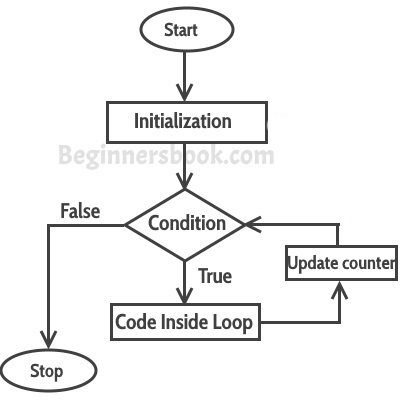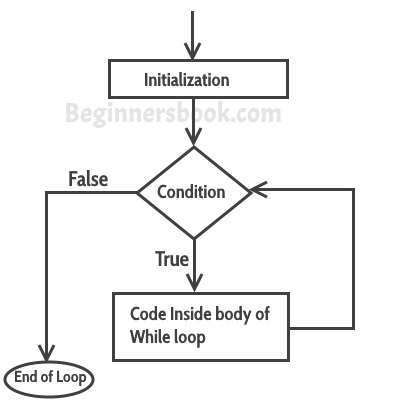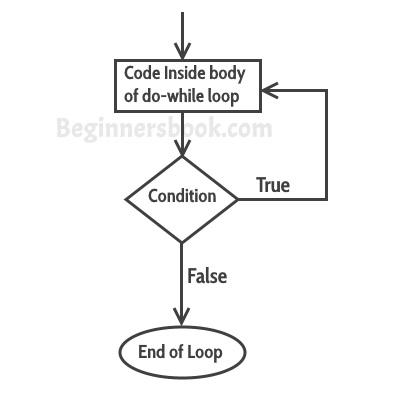Provide Best Programming Tutorials

## Java Loop Control

Loops are used to execute a set of statements repeatedly until a particular condition is satisfied.

Java has three types of loop:

• for loop
• while loop
• do…while loop

let’s talk about them one by one.

## for loop

#### Syntax of for loop:

``````for(initialization; condition ; increment/decrement)
{
statement(s);
}
``````### Example

The code below will print `0123456789`. we init a variable `counter` and give it a initial value `0`, if the `counter` is less than `10` then the code inside the curly braces will be executed.

Once the counter is not less than `10` then the loop end.

``````public static void forLoopDemo() {
for (int counter = 0; counter < 10; counter++) {
System.out.print(counter);
}
}
``````

output

`0123456789`

## while loop

#### Syntax of while loop

``````while(condition)
{
statement(s);
}
``````### Example

``````public static void whileLoopDemo() {
int counter = 0;
while (counter < 10) {
System.out.println(counter);
counter++;
}
}
``````

output:

``````1
2
3
4
5
6
7
8
9
``````

## do…while loop

#### Syntax of do-while loop:

``````do
{
statement(s);
} while(condition);
``````

### How do…while loop works

First, the statements inside loop execute and then the condition gets evaluated, if the condition returns true then the control gets transferred to the “do” else it jumps to the next statement after do-while.### Example

``````public static void doWhileLoopDemo() {
int i = 10;
do {
System.out.println(i);
i--;
} while (i > 0);
}
``````

output:

``````9
8
7
6
5
4
3
2
1
``````

Github